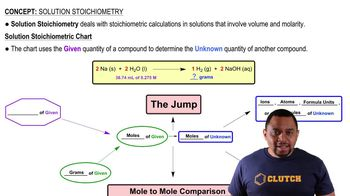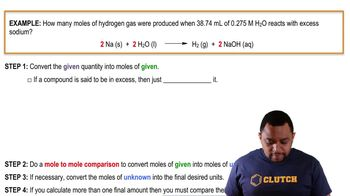Start typing, then use the up and down arrows to select an option from the list.
4:49 minutes
Problem 134
Textbook Question

# Assume that you have 1.00 g of a mixture of benzoic acid (Mol. wt. = 122) and gallic acid (Mol. wt. = 170)), both of which contain one acidic hydrogen that reacts with NaOH. On titrating the mixture with 0.500 M NaOH, 14.7 mL of base is needed to completely react with both acids. What mass in grams of each acid is present in the original mixture?Verified Solution
This video solution was recommended by our tutors as helpful for the problem above.
77views